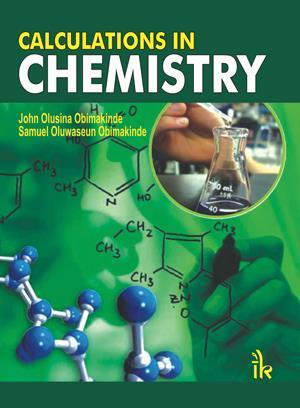John Olusina Obimakinde and Samuel Oluwaseun Obimakinde

IK International Publishing House

2015 | 722pp | £40

ISBN 9789382332732The title of this book – as well as the text on the back cover – suggests that it would teach undergraduate and postgraduate students how to undertake calculations in chemistry. Instead the book contains mostly worked calculation examples as well as numerous questions, supported by the underlying chemical principles. What is not included is any teaching of the mathematical techniques used. Students are expected to be familiar with concepts such as logarithms and calculus for example, as no further explanation of these is given. One notable exception was the integral of 1/x, which is mentioned in a footnote.

My overall impression was that of a physical chemistry textbook, in which the explanatory text has been slimmed down and the number of calculations expanded. Starting with the appropriate chemical theory, each chapter contains a number of detailed worked examples as well as practice problems and answers. The end of each chapter features multiple choice questions followed by some unfortunately named ‘essay type’ questions – the latter being actually more traditional numerical questions. These are followed by answers for both sets of questions. Unfortunately, those for the second set only consist of final answers; however, I do have some sympathy as the size of the book could grow to unmanageable proportions had more detail been included.

The core of this book are the worked examples. These are clearly presented and I found few issues with the mathematics other than those which could be put down to personal preferences. I liked the fact that in many places alternative answers were provided for the same problem.

The book provides a useful source of chemistry calculations but would need to be used in conjunction with books on physical chemistry and mathematics as appropriate for the audience.

Purchase Calculations in chemistry from Amazon.co.uk